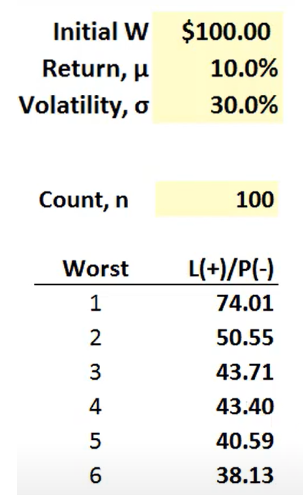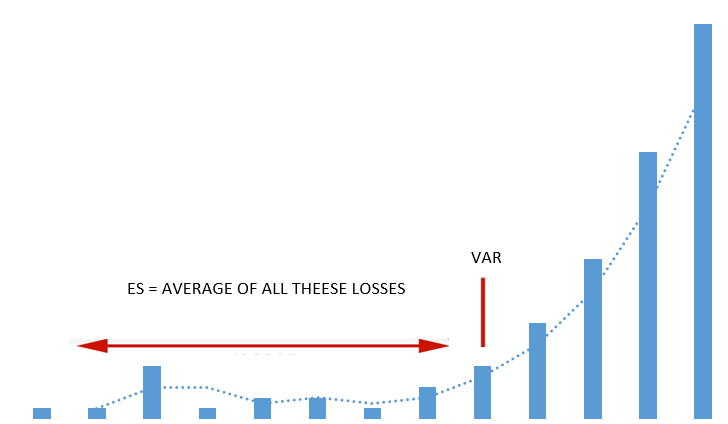Author:

Before diving into the article, let’s go ahead and define what Expected Shortfall is:

Expected Shortfall is a risk measure that indicates the average value of a possible loss in an investment that exceeds a given confidence level. It is also usually referred to as conditional value-at-risk (CVaR) and can be considered an improvement over traditional VaR when it comes to measuring risk.

## Prerequisites to understanding Expected Shortfall

The issue with CVaR is that we must first understand the basics of risk metrics and value-at-risk. Knowing the basics of these concepts will let us appreciate the reason behind CVaR’s existence and why it is a popular metric used in modern risk management.

### What are risk metrics and their characteristics?

A risk metric is a concept used for financial risk measurement to evaluate a portfolio’s market risk or credit risk. It can be thought of as the amount of cash that must be added to a position to make its risk acceptable. Expected Shortfall or conditional value at risk (CVaR) is an alternative to value at risk (VaR) and is more sensitive to the shape of the tail of the loss distribution.

There are several properties that a risk measure should have:

1. Monotonicity: if a portfolio has lower returns than another, its risk measure should be lower.
2.  Translation invariance: if we add a given amount of cash to a portfolio, its risk measure should go down according to the proportion of cash added.
3. Homogeneity: changing the size of a portfolio by a factor Y while keeping the relative amounts of different items in the portfolio the same should result in the risk measure being multiplied by Y.
4.  Sub-additivity: the risk measure for two merged portfolios should not be greater than the sum of their risk measures before joining.

We say a risk measure is coherent if it satisfies all four conditions mentioned above. The first three conditions are satisfied for VaR and CVaR, given that the risk measure is the amount of cash needed to be added to the portfolio to make its risk acceptable in the eyes of regulators. The fourth condition states that diversification helps reduce risks because when we aggregate two risks, the total risk measures corresponding to the previous ones should either decrease or stay the same.

### Basics of Value at Risk

Value at risk (VaR) is defined as the maximum possible loss over a given holding period within a fixed confidence level.

Value at risk satisfies the first three conditions but does not always satisfy the fourth. VaR also has problems measuring extreme price movements. By definition, VaR only measures the distribution quantile disregarding the severe losses beyond the VaR level so that it may ignore critical information regarding the tails of the underlying distributions. For example, imagine two hedge funds with a one-day 95% VaR of \$100. The first has its losses limited to \$105, but the second fund has no downside protection, and there is a slight chance that it can lose even more than \$500. Both funds have the same VaR, but their risk is very different.

## Expected Shortfall

Expected Shortfall or CvaR indicates the average loss when the loss exceeds the VaR level. If we are measuring VaR at the 95% confidence level, the expected Shortfall would be the average loss in the 5% cases where the fund exceeds its VaR.

As we saw in the previous sections, VaR has some shortcomings, and that’s why CVaR comes in handy. Expected Shortfall or CvaR indicates the average loss when the loss exceeds the VaR level.

If we are measuring VaR at the 95% confidence level, the expected Shortfall would be the average loss in the 5% cases where the fund exceeds its VaR. In the example above, for the fund with losses limited to \$105, and a VaR of \$100, its expected Shortfall must be between \$100 and \$105.

ES measures are susceptible to the inclusion or exclusion of very low probability extreme events, so ES measures are usually less stable than corresponding VaR measures. For example, assume two institutions agree on all scenarios, except that one says that the probability of a 100% loss is 0.1%, and the other says the probability is 0%. The two institutions will produce the same VaR but very different expected shortfalls.

ES estimates the risk of an investment in a more conservative way, focusing on the less profitable outcomes. X% ES is the expected return on the portfolio in the worst X% of cases. For small values of X, it ignores the most profitable but unlikely possibilities, while for high values of X, it focuses on the worst losses. On the other hand, unlike the discounted maximum loss, the expected Shortfall does not consider only the single most catastrophic outcome, even for higher values of X. An often-used ES is 95% ES.

As we saw from the examples above, it is clear why ES is so relevant for traders. CVaR is a measure that produces better incentives than VaR and permits more in-depth and realistic analyzes of the possible losses.

Safer investments rarely exceed VaR by a significant amount, but more volatile asset classes can exhibit CVaRs that are much greater than VaRs. Ideally, investors are looking for small values of CVaR. However, it is not uncommon for investments with the most potential to have large CVaRs.

## How to calculate Expected Shortfall (CVaR)Expected Shortfall is calculated by averaging all of the returns worse than the portfolio’s VaR at a given level of confidence.

We can see, in this example, the six worst-case scenarios out of 100 possible returns for an initial deposit of \$100. The 95% ES, in this case, is the average between cases 1, 2, 3, 4, and 5, so it is 50,45. The 97% ES is the average of the 3 worst losses because that`s the 3% tail in this case, and so on.

95% ES = AVERAGE OF 5% LOSS TAIL

99% ES = AVERAGE OF 1% LOSS TAIL

C% ES IS THE AVERAGE OF (1- C%) LOSS TAIL

In the example above, we assumed that the potential tail losses of our investment are known. It is necessary to calculate (estimate) them previously. This can be done by taking a statistical distribution or using historical data.

If you decide to use statistical distribution, you should ensure that it correctly represents the tails of your distribution. A known phenomenon is that stock returns have fat tails compared to a Gaussian distribution.

## Expected Shortfall vs. VaR (Value-at-Risk)

Expected Shortfall (CVaR) attempts to address the shortcomings of the VaR model and has been characterized as the smallest coherent and law-invariant risk measure to dominate VaR. While VaR represents a worst-case loss associated with a probability and a time horizon, CVaR represents the expected loss if that threshold is ever crossed.Although these two traders have the same VaR, trader B takes unacceptable risks. For trader B a larger loss is more likely to occur. VaR will not be able to detect those losses because it is just a number that cuts the distribution between the body and its tail. It does not take a look at what is in the tail. Expected Shortfall (ES) will look into the tail and analyze how the losses will behave if they exceed the VaR. In our case, we can observe that ES(B) > ES(A).There is another important reason for preferring ES to VaR in risk management. It is tempting for a trader to follow a nearly always profitable strategy that occasionally takes a huge blowup. An ES risk limit prevents this type of strategy, but it is possible when a VaR risk limit is used.

Because expected Shortfall is more sensitive to extreme events, there is really no way to back-test it. In general, if an investment has shown stability, then the VaR may be sufficient for risk management in that portfolio. However, the less stable the investment, the greater the chance that VaR will not give a complete picture of the risks, as it is indifferent to anything beyond its threshold. If two portfolios are combined, the total ES usually decreases, reflecting the benefits of diversification, and certainly never increases, while, in practice, the total VaR can increase.

Regulators still use VaR extensively, so its importance as a risk measure is unlikely to diminish. Expected Shortfall has several advantages over VaR, and that’s why many banks have used ES internally for years, even though only VaR is necessary to satisfy regulatory requirements. The Fundamental Review of the Trading Book (FRTB), a comprehensive suite of capital rules developed by the Basel Committee on Banking Supervision (BCBS) as part of Basel III, replaced VaR for CVaR. After about 20 years of applying value at risk with a 99% confidence level and a 10-day horizon, because of the CVaR’s ability to analyze the possible losses beyond VaR`s threshold and because of its coherency, they now apply the expected Shortfall on a 97.5% confidence level.

• Considers loss beyond the VaR level
• Better at quantifying fat tails and tail dependence
• Coherent

• almost impossible to back-test it
• Less stable than VaR

Yes, and it does satisfy the sub-additivity condition.

### Is Expected Shortfall Always Greater Than VaR?

Expected Shortfall is always a larger number than VaR because it is the average of losses that exceed the VaR.

### Is Expected Shortfall a Convex Risk Measure?

Expected Shortfall or Conditional Value-at-Risk, is a convex risk measure. The notion of convexity can replace the notions of Positive Homogeneity and Sub-additivity.

### What Does Expected Shortfall Tell Us?

To better understand, we should have in mind that, in essence, the ES answers the question: “If things do get bad, how bad can it possibly be?”

Categories:

Tags:

[convertkit form=4793161]

#### No responses yet

[convertkit form=5379902]